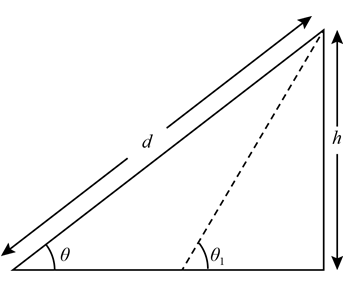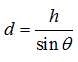×#### Thank you for registering.

One of our academic counsellors will contact you within 1 working day.

Click to Chat

1800-1023-196

+91-120-4616500

CART 0

• 0

MY CART (5)

Use Coupon: CART20 and get 20% off on all online Study Material

ITEM
DETAILS
MRP
DISCOUNT
FINAL PRICE
Total Price: Rs.

There are no items in this cart.
Continue Shopping
```
A solid wooden cylinder rolls down two different inclined planes of the same height but with different angles of inclination. Will it reach the bottom with the same speed in each case? Will it take longer to roll down one incline than the other? Explain your answers.

```
5 years ago

```							θ)/(1+(I/MR^2))Acceleration on incline plane of any rolling object can be calculated using this eqn;a = (gsinI=  moment of inertiaBoth wont  reach at same  time. You can calculate which  will reach fast using the above equation
```
5 years agoNavjyot Kalra
654 Points
```							Assume that only friction between the wooden cylinder and the inclined plane is responsible for producing the torque in the wooden cylinder, then using τ = Ia, , one can deduce the effect of angle of inclination on the motion of cylinders.Here f is the magnitude of force of friction.The diagram showing the forces acting on the wooden cylinder on inclined plane is shown below:Also, applying Newton’s third law of motion for the forces acting along the length of the inclined plane,It can be seen from this equation that the linear acceleration is directly proportional to the sine of the angle of the inclination of the inclined planeThe angular acceleration of the wooden block can be calculated using relation a = a/r, , asa = a / RIt can be seen from this equation that the angular acceleration is directly proportional to the sine of the angle of the inclination of the inclined plane.It is important to note that if the height is kept constant and the angle of inclination is changed, then the distance d (refer figure below and the dotted line) will also change, and is important to determine the time taken by the wooden cylinder to reach the bottom.The dashed line in the figure shows that changing the angle of inclination, while keeping the height constant changes the distance d to be traversed by the cylinder.The distance d can be related to the height of the inclined plane as:From this equation, one can see that if the angle θ is increases, the value of d will decrease, together with the increase in angular and linear acceleration.Therefore the wooden cylinder on inclined plane having larger angle of inclination will reach the ground earlier than the wooden cylinder on the inclined plane with smaller angle of inclination and the cylinder will have different speed when it reaches the bottom of the inclined plane.
```
5 years ago
```							a = (gsinθ)/(1+(I/MR^2)).This equation will help you sir.
```
5 years ago
Think You Can Provide A Better Answer ?

## Other Related Questions on Mechanics

View all Questions »### Course Features

• 101 Video Lectures
• Revision Notes
• Previous Year Papers
• Mind Map
• Study Planner
• NCERT Solutions
• Discussion Forum
• Test paper with Video Solution### Course Features

• 110 Video Lectures
• Revision Notes
• Test paper with Video Solution
• Mind Map
• Study Planner
• NCERT Solutions
• Discussion Forum
• Previous Year Exam Questions